### 有效利用Btree索引提高查询效率

PG的Btree索引能够处理按顺序存储的数据的=,<,>,<=,>=,以及这些操作符的等效操作，如BETWEEN，IN以及IS NULL和以字符串开头的模糊查询。

## 组合索引

tbl_index_a_b_c相当于建立了3个索引（a，b，c）、（a，b）、（a，c），看起来确实高效，但它不支持（b，c）条件组合查询。

## 用事实说话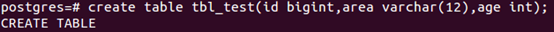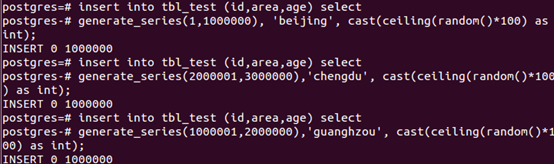• 创建索引前查询

and组合查询

``````
explain analyze  select * from tbl_test where area='beijing' and age=18;
``````or组合查询

``````
explain analyze select * from tbl_test where area='beijing' or age=18;
``````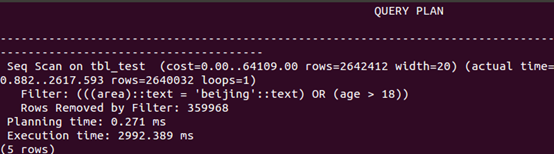``````
explain analyze select * from tbl_test where area='beijing' order by age;
``````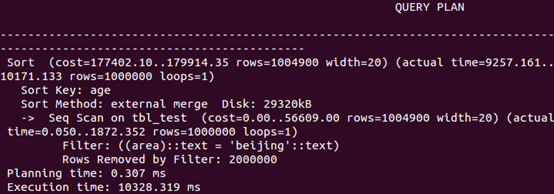• 在area和age字段上分别创建索引and组合查询

``````
explain analyze select * from tbl_test where area='beijing' and age=18;
``````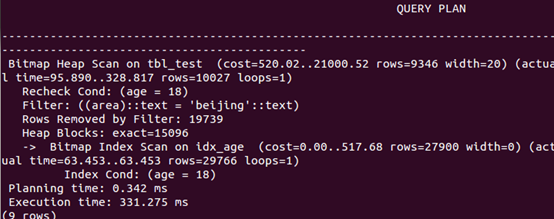or组合查询

``````
explain analyze select * from tbl_test where area='beijing' or age=18;
``````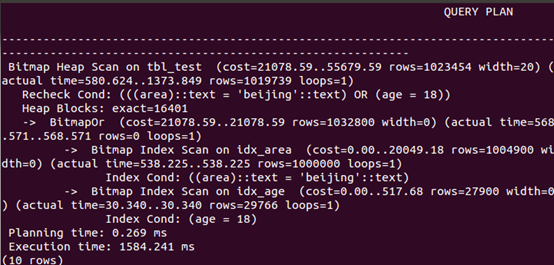``````
explain analyze select * from tbl_test where area='beijing' order by age;
``````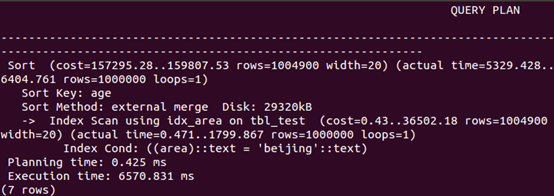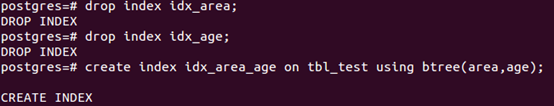• 删除单索引，在area和age字段上创建复合索引

and组合查询

``````
explain analyze select * from tbl_test where area='beijing' and age=18;
``````or组合查询

``````
explain analyze select * from tbl_test where area='beijing' or age=18;
``````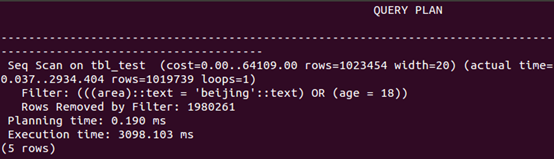``````
explain analyze select * from tbl_test where area='beijing' order by age;
``````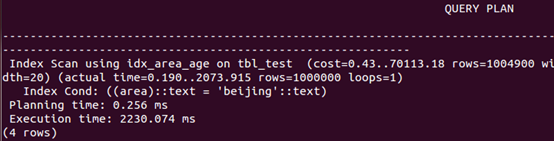``````
explain analyze select * from tbl_test where area='beijing';
````````````
explain analyze select * from tbl_test where age=18;
``````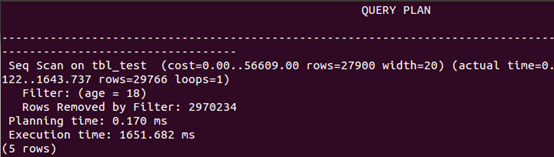## 总结：

1. 对于组合索引，一个查询可以只使用索引中的一列，但where条件中必须包含索引的第一列，即最左原则。对索引中的所有列进行搜索或前几列进行搜索时，索引非常有用。仅对后面几列进行搜索时，索引无用。
2. 对于and 组合查询，在条件字段上创建组合索引与分别创建索引相比，能带来更高的效率。
3. 对于or组合查询，创建组合索引与分别建立索引相比查询效率反而下降。
4. 对于查询中排序的字段，分别创建索引，在where子句中使用了索引，order by子句就不会使用索引。这时创建组合索引，可以大大提高查询效率。
5. 开发中，需要权衡考虑具体情况建立索引，如果创建组合索引，要仔细考虑列的顺序，将最常用做查询条件的列放在最左边，依次递减，称为最佳左前缀特性。